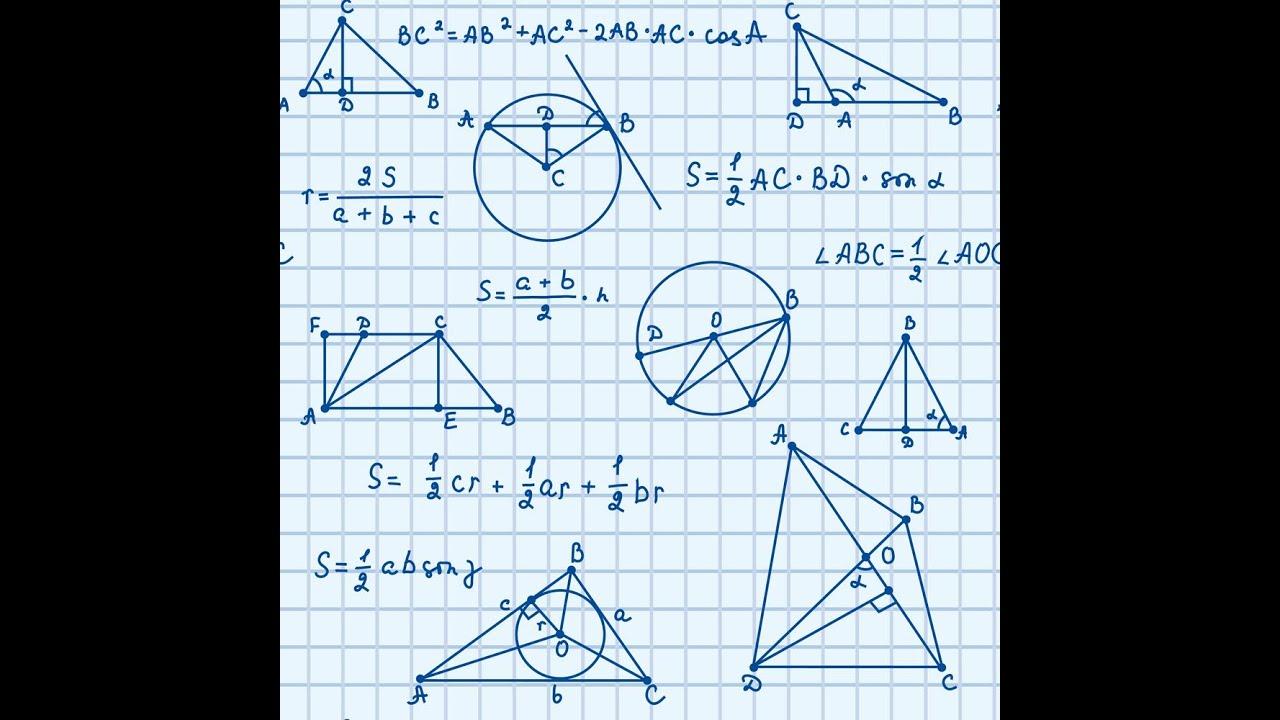• This is an overview of what we'll be covering this year!

Chapter 1 – Introduction to GeometryThe materials in this chapter introduce and cover the basics of geometry. It is organized into sections that teach, reinforce and test students on the concepts of points, lines, planes, measuring, and constructing and angle relationships.

Chapter 2 – Reasoning and Proofs
The materials in this chapter introduce and cover reasoning and proofs. It is organized into sections that teach, reinforce and test students on the concepts of logic and reasoning, classifying polygons, and proofs.

Chapter 3 – Lines and Angles
The materials in this chapter introduce and cover lines and angles. It is organized into sections that teach, reinforce and test students on the concepts of lines and angles, slopes, constructions, perpendicular lines, and midpoint and distance formulas.

Chapter 4 – Triangles I
The materials in this chapter introduce and cover triangles. It is organized into sections that teach, reinforce and test students on the concepts of classifying triangles, triangle congruence, and special parts of triangles.

Chapter 5 – Triangles II
The materials in this chapter introduce and cover triangles. It is organized into sections that teach, reinforce and test students on the concepts of indirect proofs, triangle inequalities, and similar triangles.

Chapter 6 – Polygons
The materials in this chapter introduce and cover polygons. It is organized into sections that teach, reinforce and test students on the concepts of interior angles of polygons, classifying quadrilaterals, confirming a quadrilateral is a parallelogram and similar polygons.

Chapter 7 – Area of Polygons and Circles
The materials in this chapter introduce and cover area of polygons and circles. It is organized into sections that teach, reinforce and test students on the concepts of perimeter, circumference, and area, area of polygons, perimeters and areas of similar figures, circles, arcs, and sectors and geometric probability.

Chapter 8 – Circles
The materials in this chapter introduce and cover circles. It is organized into sections that teach, reinforce and test students on the concepts of tangent lines, arcs and chords, angle relationships in circles, segment relationships in circles, equations of circles, and Reuleaux triangle.

Chapter 9 – Transformations
The materials in this chapter introduce and cover transformations. It is organized into sections that teach, reinforce and test students on the concepts of transformations and translations with matrices.

Chapter 10 – Surface Area and Volume
The materials in this chapter introduce and cover surface area and volume. It is organized into sections that teach, reinforce and test students on the concepts of surface area, volume, spheres, and volume of similar solids.